## Overview

• Whenever we discuss model prediction, it’s important to understand prediction errors (bias and variance). There is a tradeoff between a model’s ability to minimize bias and variance. Gaining a proper understanding of these errors would help us not only to build accurate models but also to avoid the mistake of overfitting and underfitting.
• So let’s start with the basics and see how they make difference to our machine learning Models.

## What is bias?

• In Data Science, bias is a deviation from expectation in the data. More fundamentally, bias refers to an error in the data. It tells you how far is your predictions from the actual values. In mathematical terms, it is the average of the difference between the actual values and the predicted values. A high bias model will give very low accuracy on both train and test data.
• Key takeaways:
• Bias is the difference between the average prediction of our model and the correct value which we are trying to predict. Model with high bias pays very little attention to the training data and oversimplifies the model. It always leads to high error on training and test data.

## What is variance?

• Variance is the variability in the model prediction-how much a ML model can adjust when we change the dataset. In a high variance model, the model tends to learn everything from the training data set. So it will give a good accuracy on the training dataset but has high error rates on the test data.
• Key takeaways:
• Variance is the variability of model prediction for a given data point or a value which tells us spread of our data. Model with high variance pays a lot of attention to training data and does not generalize on the data which it hasn’t seen before. As a result, such models perform very well on training data but has high error rates on test data.

## Mathematical Interpretation

• Let the variable we are trying to predict as $$Y$$ and other covariates as $$X$$. We assume there is a relationship between the two such that:

$Y=f(X) + e$
• where $$e$$ is the error term and it’s normally distributed with a mean of 0.
• We will make a model $$f^(X)$$ of $$f(X)$$ using linear regression or any other modeling technique.
• So the expected squared error at a point $$x$$ is:
$\operatorname{Err}(x)=E\left[(Y-\hat{f}(x))^{2}\right]$
• The $$Err(x)$$ can be further decomposed as:
$\operatorname{Err}(x)=(E[\hat{f}(x)]-f(x))^{2}+E\left[(\hat{f}(x)-E[\hat{f}(x)])^{2}\right]+\sigma_{e}^{2} \operatorname{Err}(x)= \text{Bias}^{2} + \text{Variance} + Irreducible Error$
• where $$Err(x)$$ is the sum of $$Bias^2$$, variance and the irreducible error.

• Irreducible error is the error that can’t be reduced by creating good models. It is a measure of the amount of noise in our data. Here it is important to understand that no matter how good we make our model, our data will have certain amount of noise or irreducible error that can not be removed.

## Bias and variance using bulls-eye diagram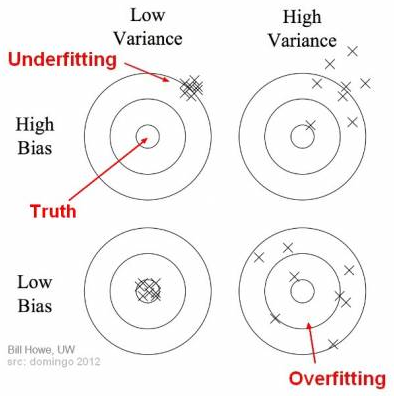• In the above diagram, center of the target is a model that perfectly predicts correct values. As we move away from the bulls-eye our predictions become get worse and worse. We can repeat our process of model building to get separate hits on the target.
• In supervised learning, underfitting happens when a model unable to capture the underlying pattern of the data. These models usually have high bias and low variance. It happens when we have very less amount of data to build an accurate model or when we try to build a linear model with a nonlinear data. Also, these kind of models are very simple to capture the complex patterns in data like Linear and logistic regression.
• In supervised learning, overfitting happens when our model captures the noise along with the underlying pattern in data. It happens when we train our model a lot over noisy dataset. These models have low bias and high variance. These models are very complex like Decision trees which are prone to overfitting.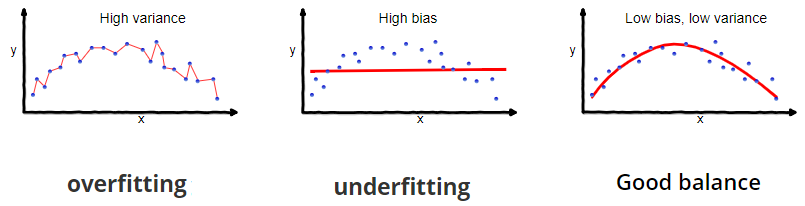## What is the Bias-Variance Tradeoff?

• The ultimate goal of any Data Scientist is to make a model that can work on multiple datasets. You need a generalized model that has low bias and low variance. It should give a good accuracy on both train and test datasets. We need to find the right/good balance without overfitting and underfitting the data.
• If our model is too simple and has very few parameters then it may have high bias and low variance. On the other hand if our model has large number of parameters then it’s going to have high variance and low bias. So we need to find the right/good balance without overfitting and underfitting the data.
• This tradeoff in complexity is why there is a tradeoff between bias and variance. An algorithm can’t be more complex and less complex at the same time.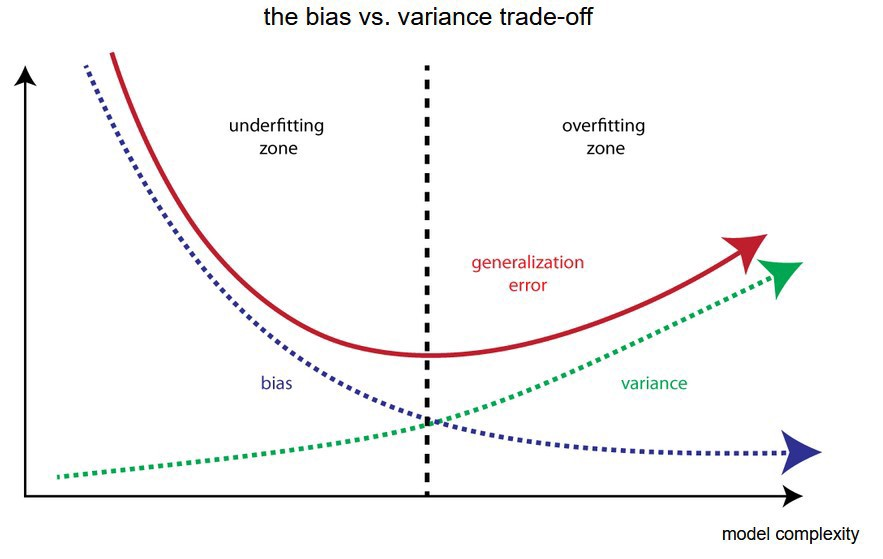• Let’s try to understand this with the help of the diagram above. We can see that as the Bias decreases the Variance increases. As that happens the complexity of the model increases and it tends to overfit the train data. We need a point where there’s a balance between the bias and variance so that the model neither underfit nor it overfits the dataset.
• Let’s understand this with an example. Suppose you need to prepare for an exam and you start preparing from the sample papers. So the sample papers would be your train data and the actual exam would be your test data. If you just learn everything from the sample papers, then you might get good accuracy on the training dataset but you might not score that much in the actual exam. It means the model is suffering from high variance. So you need to broaden your training dataset.
• But if you study from multiple sources rather than learning everything from the sample papers then there’s a higher chance of you scoring well in your exam. This is what a generalized model should be. It should give similar results on both train and test datasets.

## Total Error

• To build a good model, we need to find a good balance between bias and variance such that it minimizes the total error.
$\text { Total Error }=\text { Bias }^{\wedge} 2+\text { Variance }+\text { Irreducible Error }$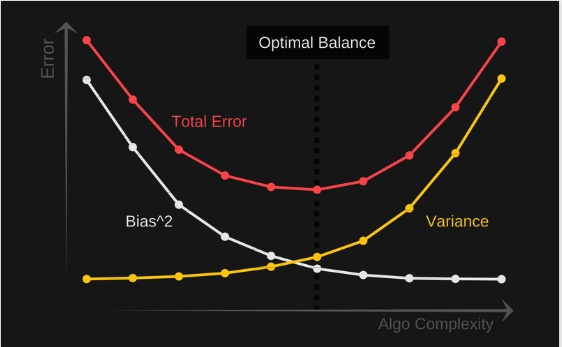• An optimal balance of bias and variance would never overfit or underfit the model.
• Therefore understanding bias and variance is critical for understanding the behavior of prediction models.

## So how do we make a balanced model?

• There are no rules to make a generalized model but there are certain things that you can do to prevent your model from overfitting or underfitting.

### Prevent overfitting

• Make sure you don’t have redundant features in your dataset.
• Use regularization (L1/L2).
• Use ensemble methods (bagging/boosting).
• Train on more data.
• Use data augmentation.
• Reduce the expressive capacity of the model.

### To prevent underfitting

• Make sure you have sufficient data.
• Make sure you have sufficient features.
• Remove outliers from the dataset.
• Increase model complexity.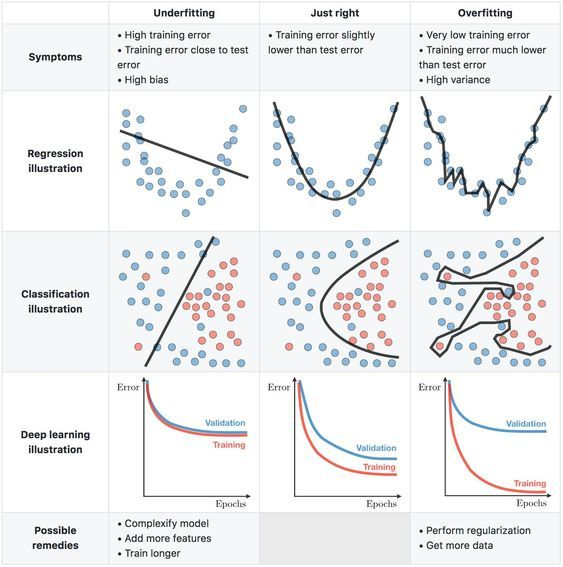## Citation

If you found our work useful, please cite it as:

@article{Chadha2020DistilledBiasVarianceTradeoff,